# A simple Bayesian model for accrual

## 2006-11-17

Suppose you are a researcher in charge of a long term study. You plan to collect data on 120 patients. The goal is to finish your study in ten years, which means getting 12 patients per year or one every thirty days on average. Recruiting patients though appears to be harder than you had expected. You recruited your first patient on day 56, 26 days behind schedule. The second patient is not recruited until day 93. About two years into the study (day 768), you have just recruited your 10th patient. It looks like recruitment might be behind schedule. Is it time to take action?

A Bayesian model of accrual times can help you to discern whether recruitment is behind schedule and project an estimated completion date allowing for uncertainty. The Bayesian model starts with an elicitation of prior beliefs about accrual rates. Then you combine the prior belief with the actual accrual data and compute a posterior distribution of accrual times. You can then use the posterior distribution to make meaningful probability statements about the current accrual rate and the projected completion time.

Elicitation of prior beliefs is difficult in most problems, but should be somewhat easier for accrual. You would not embark on a research project if you did not have at least some idea of how many patients would meet your inclusion criteria and how many would agree to participate.

You can model the accrual process as a series of waiting times between patients. A simple distribution for waiting times is the exponential distribution. The probability density function for the exponential distribution is quite simple and easy to work with.The value lambda ($$\lambda$$) of this distribution is of great interest, because it will tell you how quickly the trial will end. If lambda is equal to 30, then the average waiting time will be 30 days, exactly what you were hoping for.The 5th and 95th percentiles would be 1.5 days and 90 days respectively (see the gray shading in the picture above). This wide range is quite natural for the exponential distribution, and in many applications, the exponential distribution is a reasonably accurate approximation. If you expected to see a narrower range in waiting times, then a different distribution, such as the gamma distribution, might be a better choice.

An exponential distribution with a larger lambda value would indicate an accrual process that is much slower.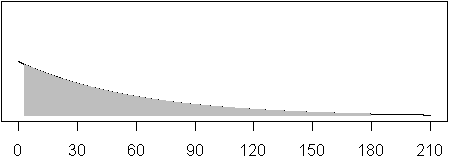Shown above is a graph of the exponential distribution with lambda=60. For this distribution, the mean is 60 days and the 5th and 95th percentiles are 3.0 and 180, respectively.

Determining the value of lambda, therefore, is critical in being able to recognize if the accrual rate is satisfactory or not. With a Bayesian model for accrual, you develop a prior distribution for lambda, and combine this with data from this clinical trial. Each time you get a new patient, you add a waiting time to the data and update the prior distribution to get a better feel for the accrual process.

There are a wide range of attractive prior distributions for accrual, but the inverse gamma distribution is attractive because of its close relationship to the exponential distribution. The probability density function for the inverse gamma distribution is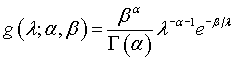The mean and variance of the inverse gamma distribution areThe second factor in the formula for the standard deviation represents the coefficient of variation (CV) also known as the relative standard deviation. Notice that as alpha increases, the CV decreases.

If you accumulate n waiting times

• x1
• x2
• xn

then the likelihood function isThe posterior distribution iswhich is proportional to an inverse gamma distribution. The parameters of the inverse gamma distribution areThe posterior mean iswhich can be written as a weighted average of the prior mean and the mean of the data.with greater weight being given to the mean of the data as the sample size increases. Let’s examine some possible prior distributions that you might use.

The value of alpha indicates the degree of certainty that you have, prior to collecting the data, about the accrual rates. Deciding how much certainty you have is a bit of a challenge, but the folks who work regularly with Bayesian models have developed a variety of approaches to eliciting this information.

Once you have specified the value for alpha, you can set the value of beta so that the expected value of the distribution is equal to your expected accrual rate (30 in this case).

If you set set alpha to a small value, such as 2, this reflects the fact that you are unable or unwilling to pin down the accrual rate. A value of beta = 30 will yield the distribution shown below.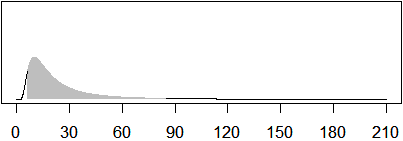The gray area represents the range of the 5th percentile (6.3 days) to the 95th percentile (84.4 days). These rates represent a broad range of accrual times, from a very fast accrual time that would allow the study to finish in an average of 2.1 years to an extremely slow accrual time that would require an astounding 28.1 years average time to completion.If you set alpha to 10 and beta to 270, you get the distribution shown above. It has the same expected value (30 days), but the 5th and 95th percentiles are closer (17.2 days and 49.8 days, respectively). These values correspond to average completion times of 5.7 years to 16.6 years.

Finally, someone who was very confident about accrual rates (perhaps because this trial is a minor variation on an existing trial) might let alpha = 50 and beta = 1470. The 5th and 95th percentiles are 23.6 days and 37.7 days, respectively. This leads to a clinical trial that would finish up, on average, in somewhere between 7.9 and 12.6 years.

Let’s choose the first prior and apply it to the existing accrual times. On day 768, you have just gotten your 10th patient. With a prior distribution with alpha = 2 and beta = 30, the posterior distribution has alpha = 2+ 10 = 12 and beta = 30 +768 = 798.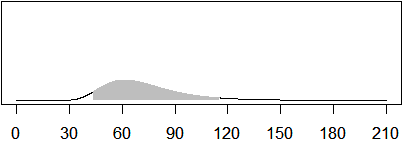With only ten patients, you do not have a very precise estimate of the accrual rate. The estimated mean accrual rate is 72.5 days. That is slightly lower than the rate based on the data alone, because the prior distribution does contain some information about the accrual rate that is incorporated into the posterior mean.

The 5th and 95th percentiles are 43.8 and 115.2 days, respectively. This is extremely wide and reminds you that we have limited data with only ten patients and limited information from the prior distribution. You do, though, have more than enough information to conclude that this trial is seriously in trouble, since even the 5th percentile is well above your target of 30 days.

Let’s choose the last prior distribution and apply it to the existing accrual times. On day 768, you have just gotten your 10th patient. Since you specified alpha = 50 and beta = 1470, you have a posterior distribution with alpha = 50+10 = 60 and beta=1470 + 768 = 2238.

Note that the average of this posterior distribution is 37.9 days and the 5th and 95th percentiles are 30.5 and 46.8 respectively. The strong prior belief outweighs the evidence from just 10 observations, but even here you can recognize problems. The 5th percentile for accrual rates is slightly above your target of 30 days in spite of your strong optimistic prior belief.

With the Bayesian models, you can also make predictions about future unobserved variables. You know that the waiting time is exponential, but you are not 100% certain about the parameter lambda in this distribution. With the first prior, it could easily be as small as 43.8 or as large as 115.2.The graph above shows what an exponential distribution looks like with lambda = 43.8, lambda = 115.2, and everything in between.

With the last prior distribution, the value of lambda could easily be as small as 30.5 or as large as 46.8.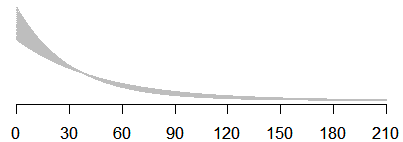The graph shown above indicates what an exponential distribution looks like with lambda = 30.5, lambda = 46.8, and everything in between.

To calculate a predictive distribution, you need to compute a weighted average of exponential distributions with weights determined by the posterior distribution. This is accomplished through a simple integration. The calculations are more tedious than difficult.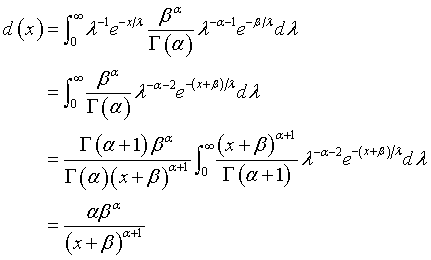The density function is closely related to a Pareto distribution. For large values of alpha, it is not too far from an exponential distribution. The expected value and standard deviation of this distribution areNotice the square root term in the standard deviation formula. This again represents the coefficient of variation. It is always larger than 1, but approaches 1 as alpha increases. The CV for an exponential distribution is exactly 1. So this formula reminds you that an exponential distribution with uncertainty in the parameter lambda leads to an increase in uncertainty.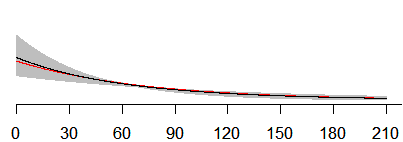The graph above shows what this predictive distribution looks like for the first prior distribution (black line). There is a small deviation from this distribution and the exponential distribution (red line).The graph above shows what the predictive distribution looks like for the last prior distribution. There is almost no visible difference between the predictive distribution and the exponential distribution.

If you assume that the remaining 110 patients accrue at an exponential rate, then the sum of these accrual times will be distributed as a gamma distribution. The density function of a gamma distribution iswhich has mean and standard deviation equal to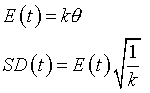Set theta to the posterior mean and k to the number of patients remaining. With the first prior distribution, set theta = 72.5 and k = 110. The mean of the remaining time in the study is 7,975 days (21.8 years) and the standard deviation is 760.4 days or 2.1 years. The 5th and 95th percentiles are 18.5 years and 25.4 years respectively.For the last prior distribution, the predictive distribution for the remainder of time would have theta = 39.7 and k = 110.The 5th and 95th percentiles are 9.7 and 13.3 years. Since we have already spent 2.1 years in the study, you can state that there is a fairly good chance that the full study will take at least 11.8 years rather than the planned 10 years.

If the number of patients remaining in the study is large, you could also rely on the central limit theorem. With the first prior distribution, the mean and standard deviation of the predictive distribution for a future waiting time are 72.5 and 79.4. The sum of 110 such variables would be approximately normal with mean = $$72.5 \times 110 = 7,975$$ days or 21.8 years and the standard deviation would be $$79.4 \times \sqrt{110} = 832.8$$ days or 2.3 years. The 5th and 95th percentiles of a normal distribution with this mean and variance would be 18.0 and 25.6 years, respectively.

You can find an earlier version of this page on my website.